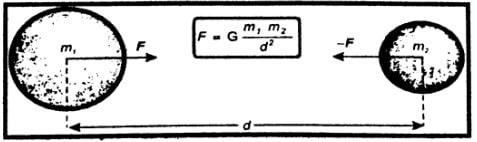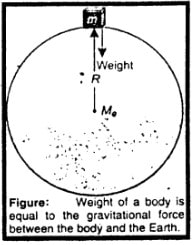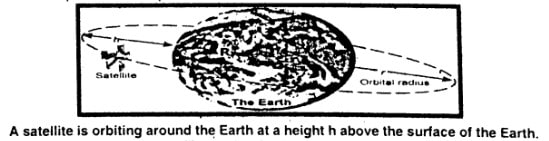What is meant by the force of gravitation?

Difficulty: Easy

The force of gravitation:

There exists a force due to which everybody in the universe attracts every other body. This force is called the force of gravitation.

Do you attract the Earth or the Earth attracts you? Which one is attracting with a larger force? You or the Earth.

Difficulty: Easy

The answer to the question can be found in two ways. First, we can use Newton’s Third Law. If object “A” exerts a force on object “B”, then object “B” will exert an equal force back on “A”. This makes it pretty clear the forces are equal.

Second, we can use Newton’s Law of Gravitational force. “The force that one mass exerts on second mass is proportional to the product of the two masses”. This means if we calculate the force the Earth exerts on us, we multiply the Earth’s mass times our mass. And if we calculate the force we exert on the Earth, we gain multiply the two masses. In other words, we do the same calculation, so we will get the same answer.

What is the field force?

Difficulty: Easy

Field force:

The gravitational pull of the Earth acting on the body whether the body is in contact with the Earth or not is called field force.

Why earlier scientists could not guess about the gravitational force?

Difficulty: Easy

Earlier scientists could not guess the force of gravitation between two masses, because it is of very small value. it could be detected only by a susceptible instrument that was not invented then.

How can you say that gravitational force is a field force?

Difficulty: Medium

The force of gravity can indeed be described as a force field. Any object having mass will create a gravitational attraction in all directions, with decreasing intensity as the distance from the object increases.

The weight of a body is due to the gravitational force with which the Earth attracts a body. Gravitational force is a non-contact force.

For example, the velocity of a body, thrown up, goes on decreasing while on return its velocity goes on increasing. This is due to the gravitational pull of the Earth acting on the body whether the body is in contact with the Earth or not. Such a force is called the field force. It is assumed that a gravitational field exists all around the Earth.

Explain, what is meant by gravitational field strength?

Difficulty: Easy

Gravitational field strength:

In the gravitational field of the Earth, the gravitational force per unit mass is called the gravitational field strength of the Earth. It is 10 N kg-1 near the surface of the Earth.

The gravitational field becomes weaker and weaker as we got farther and farther away from the Earth. At any place, its value is equal to the value of g at that point.

Why the law of gravitation is important to us?

Difficulty: Medium

Importance of law of gravitation:

As universal law of gravitation is important in releasing satellites from the earth their orbits and it also gives the reason that the y earth revolves around the sun.

The universal law of gravitation describes phenomena like the gravitational force between a planet and a star, rotation, and revolution of heavenly bodies and galaxies.

Explain Newton’s law of gravitation?

Difficulty: Medium

Law of gravitation:

Everybody in the universe attracts every other body with a force that is directly proportional to the product of their masses and inversely proportional to the square of the distance between their centers.

Explanation:

Consider two bodies of masses m1 and m2. The distance between the centers of masses is d.According to the law of gravitation, the gravitational force of attraction F with which the two masses m1 and m2 separated by a distance d attract each other is given by:

$F \: \alpha \: m_{1}m_{2}$   ……………   (i)

$F \alpha\frac{1}{d^{2}}$……………   (ii)

By combining (i) and (ii) we get

$F \: \alpha \:\frac{m_{1}m_{2}}{d^{2}}$

or

$F=G \: \frac{m_{1}m_{2}}{d^{2}}$

The universal constant of gravitation (G):

Here G is the proportionality constant. It is called the universal constant of gravitation. Its value is the same everywhere.

In SI units the value of G is  $6.673\times10^{-11}\: Nm^2{kg^{-2}}$

How the mass of the Earth can be determined?

Difficulty: Medium

Mass of the earth:

Consider a body of mass m on the surface of the Earth. Let the mass of the Earth be Me and the radius of the Earth be R. According to the law of gravitation, the gravitational force F of the Earth acting on a the the the the the the body is given by$F=G \: m \frac{Me}{R^{2}}$ ………….  (i)

But the force with which Earth attracts a body

towards its center is equal to its weight W.

Therefore,

F = W = mg

$mg= \:G \: m \frac{Me}{R^{2}}$

$g= \:G \: \frac{Me}{R^{2}}$

and   $Me=R^{2}\frac{g}{G}$

Mass $M\epsilon$ of the Earth can be determined on putting the values in equation

$Me=\left(6.4\times10^{6}m\right)^{2}\times\frac{10ms^{-2}}{6.673}\times10^{-11}Nm^{2}kg^{-2}$
$=6.0\times10^{24}kg$

Thus, the mass of the Earth is  $=6.0\times10^{24}kg$

Why does the value of g vary from place to place? Explain how the value of g varies with altitude.

Difficulty: Medium

Variation of g with altitude:

The value of g is inversely proportional to the square of the radius of the Earth

$\left(g \: a\frac{1}{R^{2}}\right)$

But it does not remain constant. It decreases with altitude. Altitude is the height of an object or place above sea level. The value of g is greater at sea level than at the hills.

Variation of g with altitude:

Equation g = G $\: \frac{me}{R^{2}}$ shows that the value of acceleration due to gravity g depends on the radius of the Earth at its surface.The value of g is inversely proportional to the square of the radius of the Earth $\left(g \: a\frac{1}{R^{2}}\right)$. But it does not remain constant. It decreases with altitude. Altitude is the height of an object or place above sea level. The value of g is greater at sea level than at the hills.

Explanation:

Consider a body of mass m at an altitude h. The distance of the body from the center of the Earth becomes R+h. Therefore, using equation g = G $\: \frac{me}{R^{2}}$ ………. (i)Note:

According to the above equation, we come to know that at a height equal to one Earth radius above the surface of the Earth. g becomes one-fourth (1/4) of its value on the Earth.

Similarly, at a distance of two Earth's radii above the Earth’s surface, the value of g becomes one-ninth (1/9) of its value on the Earth.

Can you determine the mass of our moon? If yes, then what do you need to know?

Difficulty: Medium

Yes, we can find the mass of the moon by using the law of gravitation.

$Mm=R^{2}\frac{gm}{G}$

Where Mm = mass of moon

$g_{m}$= gravitational acceleration on moon

G = gravitational constant = $6.673\times10^{-1} Nm^{2}kg^{-2}$

Explain the variation of ‘g’ with altitude.

OR

What is the effect of the following on the gravitational acceleration?

1. Mass of a freely falling body.
2. Distance of freely falling body from the center of the Earth.
3. Is there any difference between the values of g at the equator and the poles? Explain.
Difficulty: Hard

(a)      Since g = $G \: \frac{me}{R^{2}}$ …….. (i)

Equation (i) shows that the value of g does not depend upon the mass of the body. This means that light and heavy bodies should fall toward the center of the Earth with the same acceleration.

(b)      The value of g varies inversely as the square of the distance i.e. $g \: \alpha \frac{1}{R^{2}}$ if the distance from the center of the earth is increased then the value of g will decrease. That is why the value of g at hills (Murree) is less than its value of the seashore (Karachi).

(c)       Earth is not a perfect sphere. It is flattened at the poles, for this reason, the value of g at the pole is more than at the equator. Because the polar radius is less than the equatorial radius.  $g \: \alpha \frac{1}{R^{2}}$

MINI EXERCISE

1. Does an apple attract the Earth towards it?

Ans:    Yes, according to the law of gravitation an apple attracts Earth towards it but its attraction is very small and cannot be felt.

1. With what force does an apple weighing 1N attract the Earth?

Ans:    The force of attraction is equal to the weight of the object. So, an apple weighing 1N attracts the Earth with 1N force.

1. Does the weight of an apple increase, decrease, or remain when taken to the top of a mountain?

Ans:    The value of g varies inversely as the square of the distance i.e. g  1/ R2

Therefore, the weight of an apple decreases when taken to the top of a mountain due to the less gravity on Earth.

DO YOU KNOW?

The value of g on the surface of a celestial object depends on its mass and its radius. The radius of g on some of the objects is given below:

 Object g(ms-2) Sun 274.2 Mercury 3.7 Venus 8.87 Moon 1.62 Mars 3.73 Jupiter 25.94

What are artificial satellites?

Difficulty: Medium

Satellites:

An object that revolves around a planet is called a satellite. The moon revolves around the Earth so the moon is a natural satellite of the Earth.

Artificial Satellites:

Scientists have sent many objects into space. Some of these objects revolve around the Earth. These are called artificial satellites.

Uses of Artificial Satellites:

Most of the artificial satellites orbiting around the Earth are used for communication purposes. Artificial satellites carry instruments or passengers to experiment with space.Communication satellites take 24 hours to complete their one revolution around the Earth.

How Newton’s law of gravitation helps in understanding the motion of satellites? On what factors the orbital speed of a satellite depends?

OR

Derive the formula for the orbital speed of an artificial satellite?

Difficulty: Hard

The motion of Artificial Satellites:

A satellite requires a centripetal force that keeps it to move around the Earth. The gravitational force of attraction between the satellite and the earth provides the necessary centripetal force.

Consider a satellite of mass m revolving around the Earth at an altitude h in an orbit of radius  $r\circ$ with velocity vo. The necessary centripetal force required is given by equation $F\circ=m \: \frac{v\circ^{2}}{r\circ}$ ……..   (i)

This force is provided by the gravitational force of attraction between the Earth and the satellite and is equal to the weight of the satellite $w'=mg_{h}$…..   (ii)

From (i) and (ii) we get

$mg_{h}m\frac{v\circ}{r\circ}$

or    $v\circ^{2}= g_{h}r\cir$

or    $v\circ=\surd g_{h}r_{\circ}$ .......(iii)

As  $r\circ=R+h$

$v\circ= \surd g_{h\left(R+h\right)}$ ........(iv)

Equation (iii) gives us the velocity, which a satellite must possess when launched in an orbit of radius $r\circ=\left(R+h\right)$ around the Earth.

An approximation can be made for a satellite revolving close to the Earth such that R >> h.

$R+h\approx R$

And $g_{h}\approx g$

v_{\circ}=\surd gR ……..(v)

A satellite revolving around very close to the Earth has speed $v_{\circ}$ nearly 8kms^{-1}or \: 29000 \: kmh^{-1}

On what factors the orbital speed of a satellite depends?

Difficulty: Easy

Since orbital speed $=v\circ=\surd g_{h}\left(R+h\right)$

The formula shows that the orbital speed of a satellite depends upon g, R, and h.

The orbital velocity of the satellite depends on its altitude above Earth. The nearer the Earth, the faster the required orbital velocity.

Why are communication satellites stationed in geostationary orbits?

Difficulty: Easy

Communication satellites and weather satellites are often given geostationary orbits, so that the satellite antennas that communicate with them do not have to move to track them, but can be pointed permanently at the position in the sky where they stay. A geostationary orbit is a particular type of geosynchronous orbit.